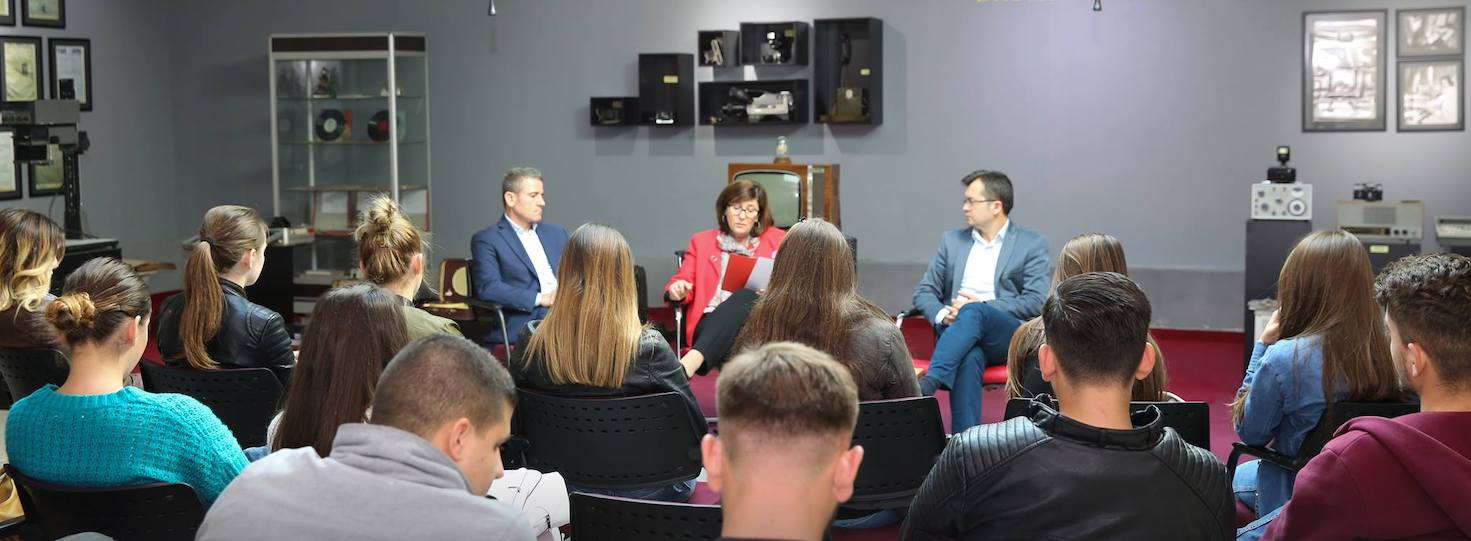Course Title : Statistics for Social Sciences
Code Course
Type
Regular
Semester
Lecture
(hours/week)
Seminar
(hours/week)
Lab
(hours/week)
Credits ECTS
SOC 202-1 A 4 3 0 0 3.00 5
Lecturer and Office Hours
Teaching Assistant and Office Hours
Language Albanian
Course Level
Description This module aims to give students the basic knowledge in statistics and to help them in a properly usage of the statistical concepts and techniques correlated with other courses and practice work they are undertaking. The course is focused in a combination of theoretical aspects with the practical ones, trying to improve students’ capacity in implementing efficient use of statistical analysis. This module addresses the basic concepts of statistics which serve as a support point for the recognition and use of other statistical concepts that are based on them.
Objectives The course aims to provide students: 1. the opportunity to understand the importance of statistics for social sciences 2. the ability to efficiently apply the statistical methods in different situations in the social sciences 3. the knowledge on how to gather, organize, process, analyze and interpret the data. 4. the capacity to identify appropriate statistical techniques for data analysis in the field of social sciences.
Course Outline
WeekTopics
1Data, measurement and statistics.
2Descriptive statistics, tabular and graphical methods.
3Localization and variation indicators.
4Introduction to probability.
5Probability distributions of discrete random variables.
6Probability distribution of continues variables.
7Sampling, Sampling distribution and confidence interval.
8Midterm Exam
9Hypotheses testing for the population mean and proportions.
10Hypotheses testing for 2 population means and proportions.
11Hypotheses testing for the population variance.
12Good and fit test.
13Analyze of Variance.
14Simple linear regression and correlation.
15Multiple linear regression.
16Final Exam
Prerequisites
Textbook
Other References
Laboratory Work
Computer Usage
Other
Learning Outcomes and Competences
1Students will gain good knowledge of the statistical concepts and techniques.
2Students will obtain capability to apply the statistical modeling in social sciences.
3Students will be capable to analyze and interpret the results of statistical output.
Course Evaluation Methods
In-term studies Quantity Percentage
Midterms130
Quizzes220
Projects00
Term Projects00
Laboratory00
Attendance110
Contribution of in-term studies to overall grade60
Contribution of final examination to overall grade40
Total100
ECTS (Allocated Based on Student) Workload
Activities Quantity Duration
(hours)